# Homotopy type of connected sum depends on choice of gluing map

## Statement

It is possible to find an example of compact connected orientable manifolds$M_1$ and$M_2$ such that the homotopy type of the connected sum$M_1 \# M_2$ is not well defined, i.e., we can get connected sums of different homotopy types depending on the choice of the gluing map.

## Facts used

1. Complex projective space has orientation-reversing self-homeomorphism iff it has odd complex dimension

## Proof

To construct an example, we need to find a case where both$M_1$ and$M_2$ are orientable but neither of them has an orientation-reversing self-homeomorphism. One simple choice, by Fact (1), is to set both$M_1$ and$M_2$ as homeomorphic to the complex projective plane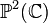$\mathbb{P}^2(\mathbb{C})$ which has real dimension 4.

There are two possible connected sums:

• Connected sum of two complex projective planes with same orientation: This has cohomology ring isomorphic to$\mathbb{Z}[x,y]/(x^2 - y^2,x^3,y^3)$, where$x,y$ are additive generators of the free abelian group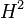$H^2$ and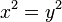$x^2 = y^2$ is the additive generator for$H^4$.
• Connected sum of two complex projective planes with opposite orientation: This has cohomology ring isomorphic to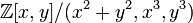$\mathbb{Z}[x,y]/(x^2 + y^2,x^3,y^3)$, where$x,y$ are additive generators of the free abelian group$H^2$ and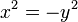$x^2 = -y^2$ is the additive generator for$H^4$.